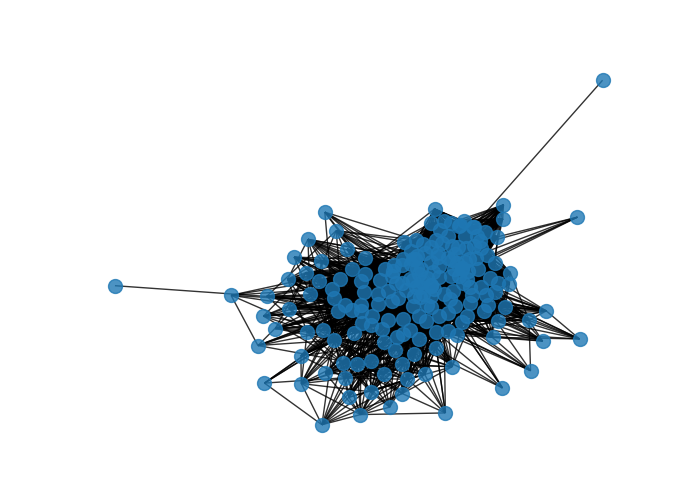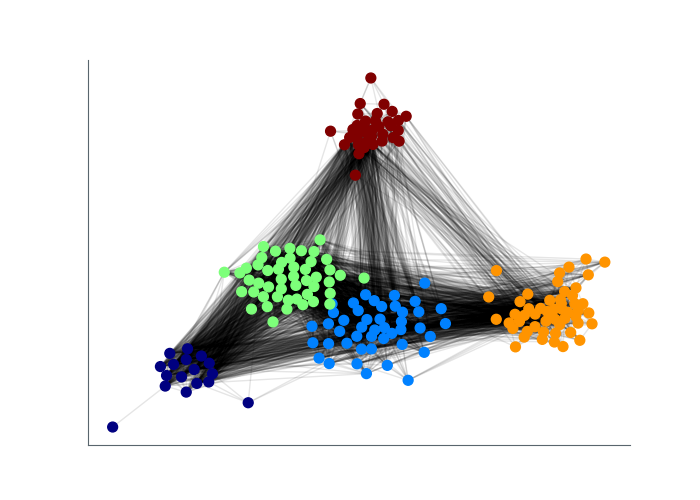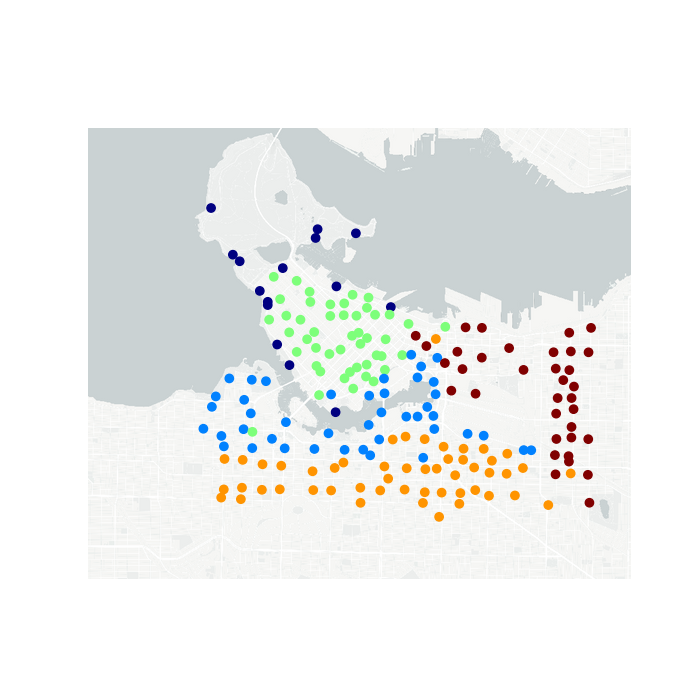# Mobi Station Networks

I've started wondering if there are any network effects in Mobi trip data. I don't know much about network analysis, but luckily there are python packages to do the work for me: NetworkX provides general network analysis tools and basic plotting, and Community provides tools for determining clusters in a network. In this post, I'll use these tools to investigate how stations cluster together based on the frequency of trips between pairs of stations

### Data prep¶

Data for this post comes from Mobi Bikes' official system data. I've done some minimal cleaning to consolidate the data and drop spurious records. To make the data a bit more manageable, I'll only look at the summer of 2019, May through August (inclusive).

In :
import mobisys
import pandas as pd
import networkx as nx
import matplotlib.pyplot as plt
import community
import numpy as np

import cartopy.crs as ccrs
from credentials import MAPBOX_TOKEN
from shapely.geometry import Point
import geopandas

In :
%matplotlib notebook

In :
fulldf = pd.read_csv('/home/msj/mobi-dash/data/Mobi_System_Data_Prepped_full.csv',index_col=0,parse_dates=True)

/home/msj/miniconda3/lib/python3.7/site-packages/IPython/core/interactiveshell.py:3049: DtypeWarning: Columns (2) have mixed types. Specify dtype option on import or set low_memory=False.
interactivity=interactivity, compiler=compiler, result=result)

In :
df = fulldf['2019-05':'2019-08']


Next, I sum up the trips based on the Departure Station and Return Station. This gives us a table with one record for each pair of stations, with the number of trips taken between those stations.

In :
cdf = df.groupby(['Departure station','Return station']).size()
cdf = cdf.reset_index()
cdf.columns = ['Departure station','Return station','trips']
cdf = cdf.sort_values('trips',ascending=False)

In :
cdf.head()

Out:
Departure station Return station trips
23855 Stanley Park - Information Booth Stanley Park - Information Booth 3231
24328 Stanley Park - Totem Poles Stanley Park - Second Beach North 3177
23859 Stanley Park - Information Booth Stanley Park - Totem Poles 2893
23856 Stanley Park - Information Booth Stanley Park - Second Beach North 1742
24331 Stanley Park - Totem Poles Stanley Park - Totem Poles 1557

### Build the network¶

Now it's time to make the network. Luckily, NetworkX provides a convenience function to create a network object froma pandas dataframe. I just need to specify that the Departure station and Return station correspond to the nodes, and trips corresponds to the edges. In the interest of processing time, I'll only use connections that have had at least 10 trips between nodes and drop the rest.

In :
G=nx.from_pandas_edgelist(cdf[cdf.trips>10], 'Departure station', 'Return station', 'trips')


We can try drawing this network, but right now it's not going to show us anything interesting. Most stations have connections to most other stations, so the network just looks like a mass of well-connected nodes.

In :
f,ax = plt.subplots()
nx.draw(G,alpha=0.8, node_size=100)### Partition the network¶

One way to look for internal structure in the network is the Louvain method for community detection, which is implemented by the python package community as the function best_partition(). According to the docs:

Compute the partition of the graph nodes which maximises the modularity
(or try..) using the Louvain heuristices

This is the partition of highest modularity, i.e. the highest partition
of the dendrogram generated by the Louvain algorithm.

This function creates a dictionary that labels each node as part of a community according to the Louvain algorithm.

In :
partition = community.best_partition(G,weight='trips')

In :
set(partition.values())

Out:
{0, 1, 2, 3, 4}

We see that the algorithm has divided our network into 5 communities. Let's visualize these communities on a network graph. I've added some helper functions to position the different communities in clusters. Node that in these visualizations the length of the edge isn't necessarily proportional to the strength of the connection.

In :
#https://stackoverflow.com/questions/43541376/how-to-draw-communities-with-networkx

import numpy as np
import matplotlib.pyplot as plt
import networkx as nx

def community_layout(g, partition):
"""
Compute the layout for a modular graph.

Arguments:
----------
g -- networkx.Graph or networkx.DiGraph instance
graph to plot

partition -- dict mapping int node -> int community
graph partitions

Returns:
--------
pos -- dict mapping int node -> (float x, float y)
node positions

"""

pos_communities = _position_communities(g, partition, scale=3.)

pos_nodes = _position_nodes(g, partition, scale=1.)

# combine positions
pos = dict()
for node in g.nodes():
pos[node] = pos_communities[node] + pos_nodes[node]

return pos

def _position_communities(g, partition, **kwargs):

# create a weighted graph, in which each node corresponds to a community,
# and each edge weight to the number of edges between communities
between_community_edges = _find_between_community_edges(g, partition)

communities = set(partition.values())
hypergraph = nx.DiGraph()
for (ci, cj), edges in between_community_edges.items():

# find layout for communities
pos_communities = nx.spring_layout(hypergraph, **kwargs)

# set node positions to position of community
pos = dict()
for node, community in partition.items():
pos[node] = pos_communities[community]

return pos

def _find_between_community_edges(g, partition):

edges = dict()

for (ni, nj) in g.edges():
ci = partition[ni]
cj = partition[nj]

if ci != cj:
try:
edges[(ci, cj)] += [(ni, nj)]
except KeyError:
edges[(ci, cj)] = [(ni, nj)]

return edges

def _position_nodes(g, partition, **kwargs):
"""
Positions nodes within communities.
"""

communities = dict()
for node, community in partition.items():
try:
communities[community] += [node]
except KeyError:
communities[community] = [node]

pos = dict()
for ci, nodes in communities.items():
subgraph = g.subgraph(nodes)
pos_subgraph = nx.spring_layout(subgraph, **kwargs)
pos.update(pos_subgraph)

return pos

In :
pos = community_layout(G, partition)
colors = [partition[x] for x in G.nodes]

f,ax = plt.subplots()
nx.draw_networkx_nodes(G, pos, node_color=colors,node_size=50,alpha=1,cmap='jet')
nx.draw_networkx_edges(G,pos,alpha=0.1)Out:
<matplotlib.collections.LineCollection at 0x7f03cb032ef0>

This is all too abstract. To make sense of these communities, let's plot the stations on a map and color them according to which community they've been sorted in to. First, I need a list of stations with their lat/long coordinates. For this I recommend my bikedata package for querying bikeshare APIs (see my previous post for details).

In :
import bikedata as bd

bs = bd.BikeShareSystem('mobi_bikes')
sdf = bs.query_station_info()

In :
sdf.head()

Out:
capacity lat lon name station_id
0 52 49.262487 -123.114397 10th & Cambie 0001
1 28 49.285871 -123.121050 Burrard Station 0002
2 16 49.274566 -123.121817 Yaletown-Roundhouse Station 0004
3 26 49.279764 -123.110154 Dunsmuir & Beatty 0005
4 26 49.266675 -123.115635 Olympic Village Station 0006

For mapping, I'll convert the pandas dataframe into a geopandas dataframe to better handle coordinate projections, and I'll add the community label created when we partitioned the network.

In :
sdf['geometry'] = [Point(xy) for xy in zip(sdf.lon, sdf.lat)]
sdf = geopandas.GeoDataFrame(sdf)
sdf.crs = {'init' :'epsg:4326'}
sdf = sdf.to_crs({'init': 'epsg:3857'})
sdf['partition'] = sdf.name.map(partition)

Out:
capacity lat lon name station_id geometry partition
0 52 49.262487 -123.114397 10th & Cambie 0001 POINT (-13705031.98336093 6319517.879010359) 3.0
1 28 49.285871 -123.121050 Burrard Station 0002 POINT (-13705772.59193318 6323507.663575065) 2.0
2 16 49.274566 -123.121817 Yaletown-Roundhouse Station 0004 POINT (-13705857.97398261 6321578.565172686) 2.0
3 26 49.279764 -123.110154 Dunsmuir & Beatty 0005 POINT (-13704559.65476149 6322465.503044237) 2.0
4 26 49.266675 -123.115635 Olympic Village Station 0006 POINT (-13705169.7711981 6320232.349643161) 1.0
In :
lon_min = -123.185
lon_max = -123.056
lat_min = 49.245
lat_max = 49.315

f,ax = plt.subplots(subplot_kw={'projection': ccrs.epsg(3857)},figsize=(7,7))
tile = MapboxStyleTiles(MAPBOX_TOKEN,'mjarrett','ck3gggjkl03gp1cpfm927yo7c')
extent = [lon_min,lon_max,lat_min,lat_max]
ax.set_extent(extent)

sdf.plot(ax=ax,column='partition', cmap='jet')

ax.outline_patch.set_visible(False)
ax.background_patch.set_visible(False)The meanings behind the community partitions are now obvious. I'll name the five communities as: